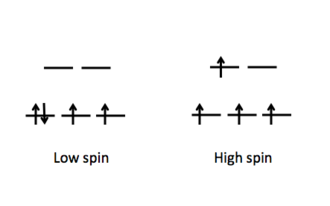# 1.11: Pi Donor and Acceptor Ligands

$$\newcommand{\vecs}{\overset { \rightharpoonup} {\mathbf{#1}} }$$ $$\newcommand{\vecd}{\overset{-\!-\!\rightharpoonup}{\vphantom{a}\smash {#1}}}$$$$\newcommand{\id}{\mathrm{id}}$$ $$\newcommand{\Span}{\mathrm{span}}$$ $$\newcommand{\kernel}{\mathrm{null}\,}$$ $$\newcommand{\range}{\mathrm{range}\,}$$ $$\newcommand{\RealPart}{\mathrm{Re}}$$ $$\newcommand{\ImaginaryPart}{\mathrm{Im}}$$ $$\newcommand{\Argument}{\mathrm{Arg}}$$ $$\newcommand{\norm}{\| #1 \|}$$ $$\newcommand{\inner}{\langle #1, #2 \rangle}$$ $$\newcommand{\Span}{\mathrm{span}}$$ $$\newcommand{\id}{\mathrm{id}}$$ $$\newcommand{\Span}{\mathrm{span}}$$ $$\newcommand{\kernel}{\mathrm{null}\,}$$ $$\newcommand{\range}{\mathrm{range}\,}$$ $$\newcommand{\RealPart}{\mathrm{Re}}$$ $$\newcommand{\ImaginaryPart}{\mathrm{Im}}$$ $$\newcommand{\Argument}{\mathrm{Arg}}$$ $$\newcommand{\norm}{\| #1 \|}$$ $$\newcommand{\inner}{\langle #1, #2 \rangle}$$ $$\newcommand{\Span}{\mathrm{span}}$$

The nature of ligands coordinated to the center metal is an important feature of a complex compound along with other properties such as metal identify and its oxidation state. More specifically, it is the identity and consequently the ability of the ligand to donate or accept electrons to the center atom that will determine the molecular orbitals.

The spectrochemical series shows the trend of compounds as weak field to strong field ligands. Furthermore, ligands can be characterized by their π-bonding interactions. This interaction reveals the amount of split between eg and t2g energy levels of the molecular orbitals that ultimately dictates the strength of field of the ligands.

Examples of Weak Field Ligands X-, OH-, H2O ; Examples of Strong Field Ligands H-, NH3, CO, PR3Figure $$\PageIndex{1}$$: MO Diagrams of Pi Donor Ligands and Pi Acceptor Ligands.Figure $$\PageIndex{2}$$: Electron configuration of high and low spin.

Electron configuration of high and low spin.

In a π-donor ligand, the SALCs of the ligands are occupied, hence it donates the electrons to the molecular σ σ* and π π* orbitals. The orbitals associated to eg are not involved in π interactions therefore it stays in the same energy level (Figure $$\PageIndex{1}$$). On the other hand, the occupied ligand SALC t2g orbitals that would form molecular orbitals with the metal t2g orbitals (ie. dxy, dxz, dyz) are lower in energy than its metal counterparts. The resulting MO has π* orbitals that are energetically lower than the σ* orbitals that are formed from the non bonding orbitals (eg). The difference between the t2g π* and eg σ orbitals is denoted as Δ, split. In the π-donor case, the Δ is small due to the low π* level.

Conversely, the t2g SALCs of a pi accepting orbitals are higher in energy than the metal t2g orbitals because they are unoccupied. The resulting t2g π* orbitals are higher than the σ* orbitals. This creates a larger Δ between the eg and t2g π orbitals, making these π-accepting orbitals high split ligands.

Finally, the magnitude of Δ as influenced by the identify of the ligand will dictate how electrons are distributed in the metal d orbitals (Figure $$\PageIndex{2}$$). Weak field ligands produce a small Δ hence a high spin configuration. Strong field ligands produce a large Δ hence a low spin configuration on the d electrons.

1.11: Pi Donor and Acceptor Ligands is shared under a CC BY-SA license and was authored, remixed, and/or curated by LibreTexts.